Download the eSaral app and start learning from Kota's top IITians and doctors.

# ElectroChemistry - JEE Advanced Previous Year Questions with Solutions

JEE Advanced Previous Year Questions of Chemistry with Solutions are available at eSaral. Practicing JEE Advanced Previous Year Papers Questions of Chemistry will help the JEE aspirants in realizing the question pattern as well as help in analyzing weak & strong areas. Simulator Previous Years JEE Advance Questions
Q. For the reaction of $\mathrm{NO}_{3}^{-}$ ion in an aqueous solution, $\mathrm{E}^{\circ}$ is +0.96 V. Values of $\mathrm{E}^{\circ}$ for some metal ions are given below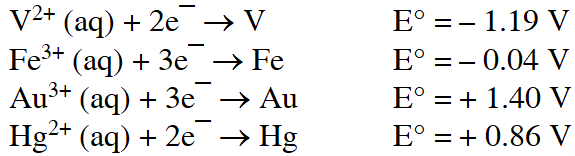The pair(s) of metal that is(are) oxidised by $\mathrm{NO}_{3}^{-}$ in aqueous solution is(are) (A) V and Hg (B) Hg and Fe (C) Fe and Au (D) Fe and V [JEE 2009]
Ans. (A,B,D) (A,B,D) as $\mathrm{E}^{\circ}$ will be positive
Paragraph for Questions 2 to 3 The concentration of potassium ions inside a biological cell is at least twenty times higher than the outside. The resulting potential difference across the cell is important in several processes such as transmission of nerve impulses and maintaining the ion balance. A simple model for such a concentration cell involving a metal M is : $\mathrm{M}(\mathrm{s}) | \mathrm{M}^{+}\left(\mathrm{aq} ; 0.05 \text { molar) } \| \mathrm{M}^{+}(\mathrm{aq} ; 1 \mathrm{molar}) | \mathrm{M}(\mathrm{s})\right.$ For the above electrolytic cell the magnitude of the cell potential $\left|\mathrm{E}_{\mathrm{cell}}\right|$ = 70 mV.
Q. For the above cell :- (A) $\mathrm{E}_{\text {cell }}<0 ; \Delta \mathrm{G}>0$ (B) $\mathrm{E}_{\text {cell }}>0 ; \Delta \mathrm{G}<0$ (C) $\mathrm{E}_{\text {cell }}<0 ; \Delta \mathrm{G}^{0}>0$ (D) $\mathrm{E}_{\text {cell }}>0 ; \Delta \mathrm{G}^{\text {o }}<0$ [JEE 2010]
Ans. (C) $\mathrm{E}_{1}=-\frac{059}{1} \log \frac{05}{1}=(+) \mathrm{ve} \Rightarrow \mathrm{so}$
Q. If the 0.05 molar solution of $\mathrm{M}^{+}$ is replaced by a 0.0025 molar $\mathrm{M}^{+}$ solution, then the magnitude of the cell potential would be :- (A) 35 mV              (B) 70 mV             (C) 140 mV              (D) 700 mV [JEE 2010]
Ans. (B)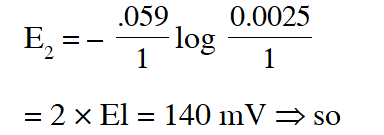Q. Consider the following cell reaction : $2 \mathrm{Fe}_{(\mathrm{s})}+\mathrm{O}_{2(\mathrm{g})}+4 \mathrm{H}_{(\mathrm{aq})}^{+} \rightarrow 2 \mathrm{Fe}_{(\mathrm{aq})}^{2+}+2 \mathrm{H}_{2} \mathrm{O}(\ell) \quad \mathrm{E}^{\circ}=1.67 \mathrm{V}$ $\mathrm{At}\left[\mathrm{Fe}^{2+}\right]=10^{-3} \mathrm{M}, \mathrm{P}\left(\mathrm{O}_{2}\right)=0.1$ atm and $\mathrm{pH}=3,$ the cell potential at $25^{\circ} \mathrm{C}$ is – (A) 1.47 V (B) 1.77 V (C) 1.87 V (D) 1.57 V [JEE 2011]
Ans. (D)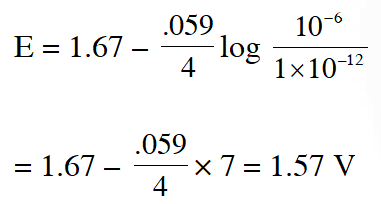Q. $\mathrm{AgNO}_{3}$ (aq.) was added to an aqueous KCl solution gradually and the conductivity of the solution was measured. the plot of conductance () versus the volume of $\mathrm{AgNO}_{3}$ is -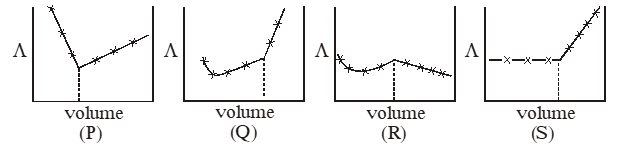(A) (P) (B) (Q) (C) (R) (D) (S) [JEE 2011]
Ans. (D)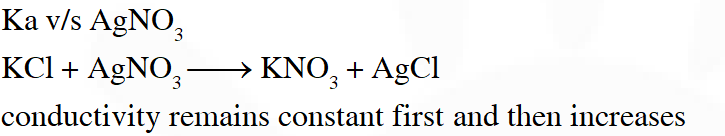Paragraph for Question 6 and 7 The electrochemical cell shown below is a concentration cell. $\mathrm{M} | \mathrm{M}^{2+}$ (saturated solution of a sparingly soluble salt, $\mathrm{MX}_{2}$) | | $\mathbf{M}^{2+}$ (0.001 mol $\mathrm{dm}^{-3}$) | M The emf of the cell depends on the difference in concentrations of M2+ ions at the two electrodes. The emf of the cell at 298 K is 0.059V.
Q. The value of G $\left(\mathrm{kJ} \mathrm{mol}^{-1}\right)$ for the given cell is (take If = 96500 C $\mathrm{mol}^{-1}$) (A) –5.7 (B) 5.7 (C) 11.4 (D) –11.4. [JEE 2012]
Ans. (B)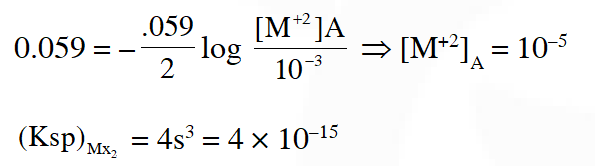Q. The solubility product $\left(\mathrm{K}_{\mathrm{sp}} ; \mathrm{mol}^{3} \mathrm{dm}^{-9}\right)$ of $\mathrm{MX}_{2}$ at 298 K based on the information available for the given concentration cell is (take 2.303 × R × 298/F = 0.059 V) (A) $1 \times 10^{-15}$ (B) $4 \times 10^{-15}$ (C) $1 \times 10^{-12}$ (D) $1 \times 10^{-12}$
Ans. (D) $\Delta \mathrm{G}=\frac{-2 \times 96500 \times .059}{1000}=11.4$
Q. The standard reduction potential data at $25^{\circ} \mathrm{C}$ is given below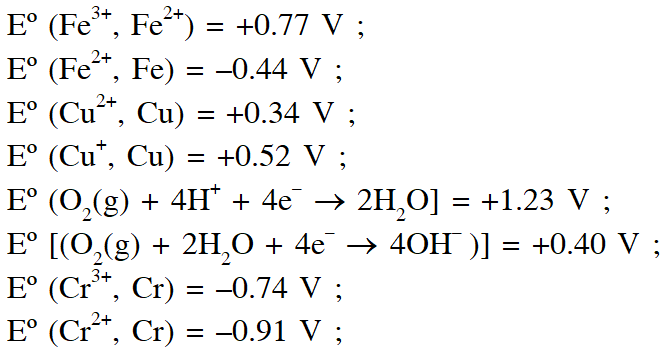Match $\mathrm{E}^{\mathrm{o}}$ of the redox pair in List-I with the values given in List-II and select the correct answer using the code given below the lists :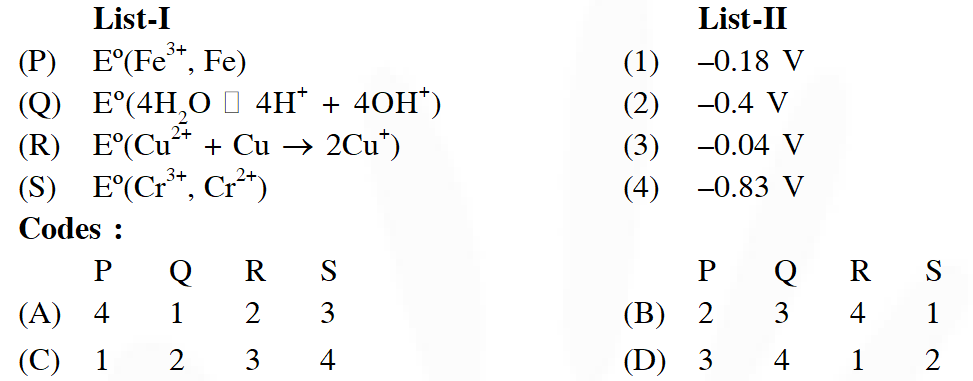[JEE-Adv. 2013]
Ans. (D)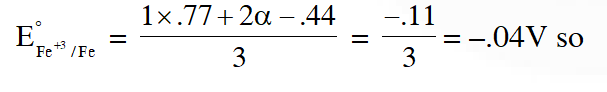Q. An aqueous solution of X is added slowly to an aqueous solution of Y as shown in List-I. The variation in conductivity of these reactions is given in List-II. Match List-I with List-II and select the correct answer using the code given below the lists :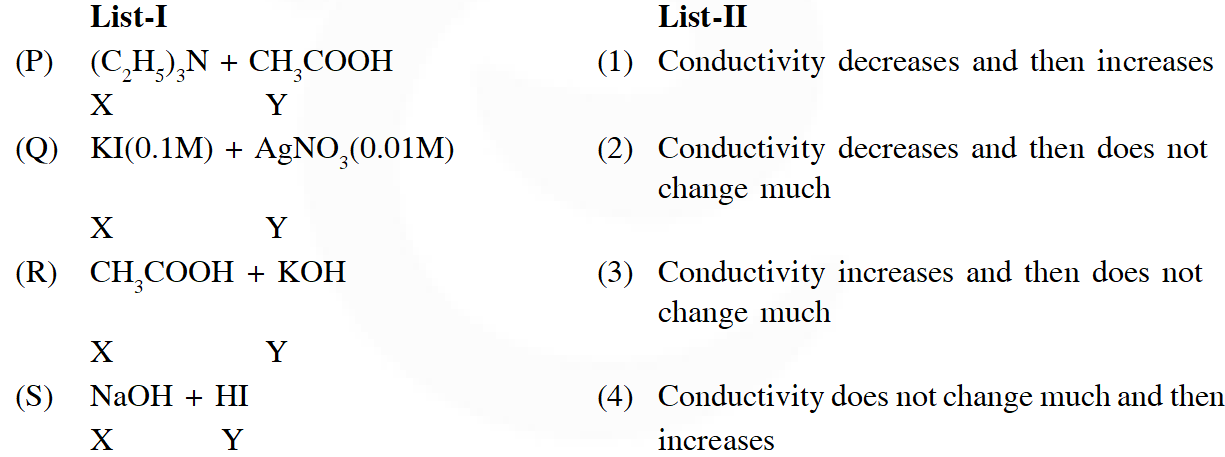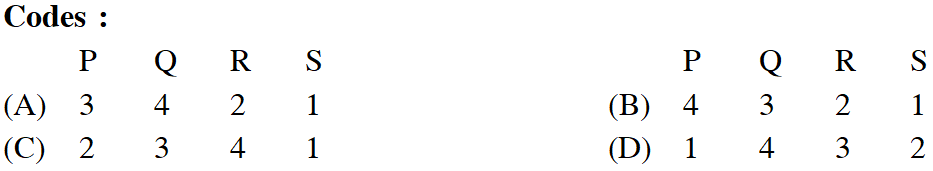[JEE-Adv. 2013]
Ans. (A) $\left(\mathrm{C}_{2} \mathrm{H}_{5}\right)_{3} \mathrm{N}+\mathrm{CH}_{3} \mathrm{COOH} \Rightarrow$ Weak acid and weak base so conductivity increases and then does not change much so option 3 hence and (a)
Q. In a galvanic cell , the salt bridge - (A) Does not participate chemically in the cell reaction (B) Stops the diffusion of ions from one electrode to another (C) Is necessary for the occurence of the cell reaction (D) Ensures mixing of the two electrolytic solutions [JEE-Adv. 2014]
Ans. (A,B) Fact
Q. The molar conductivity of a solution of a weak acid HX (0.01 M) is 10 times smaller than the molar conductivity of a solution of a weak acid HY (0.1 M). If $\lambda_{\mathrm{X}^{-}}^{0} \approx \lambda_{\mathrm{Y}^{-}}^{0}$ the difference in their $\mathrm{pK}_{\mathrm{a}}$ values $, \mathrm{pK}_{\mathrm{a}}(\mathrm{HX})-\mathrm{p} \mathrm{K}_{\mathrm{a}}(\mathrm{HY}),$ is (consider degree of ionization of both acids to be <<1). [JEE-Adv. 2015]
Ans. 3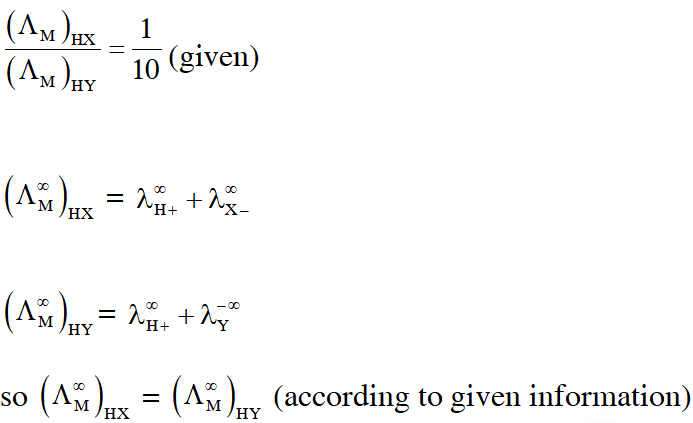Q. All the energy released from the reaction X $\rightarrow \mathrm{Y}, \Delta_{\mathrm{r}} \mathrm{G}^{\circ}=-193 \mathrm{kJ} \mathrm{mol}^{-1}$ is used for the oxidizing $\mathrm{M}^{+}$ and $\mathrm{M}^{+} \rightarrow \mathrm{M}^{3+}+2 \mathrm{e}^{-}, \mathrm{E}^{\circ}=-0.25 \mathrm{V}$ Under standard conditions, the number of moles of M+ oxidized when one mole of X is converted to Y is $\left[\mathrm{F}=96500 \mathrm{C} \mathrm{mol}^{-1}\right]$ [JEE-Adv. 2015]
Ans. 4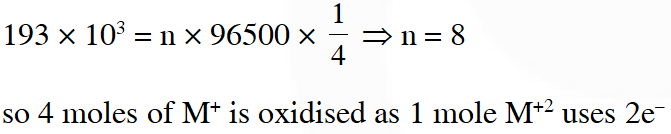Q. For the electrochemical cell, $\mathrm{Mg}(\mathrm{s})\left|\mathrm{Mg}^{2+}(\mathrm{aq}, 1 \mathrm{M}) \| \mathrm{Cu}^{2+}(\mathrm{aq}, 1 \mathrm{M})\right| \mathrm{Cu}(\mathrm{s})$ the standard emf of the cell is 2.70 V at 300 K. When the concentration of $\mathrm{Mg}^{2+}$ is changed to x M, the cell potential changes to 2.67 V at 300 K. The value of x is____. (given, $\frac{\mathrm{F}}{\mathrm{R}}=11500 \mathrm{KV}^{-1}$ where F is the Faraday constant and R is the gas constant, ln(10) = 2.30) [JEE-Adv. 2018]
Ans. 10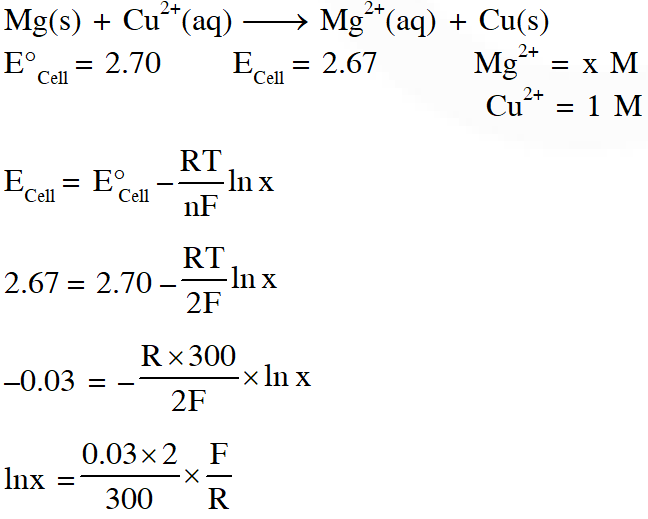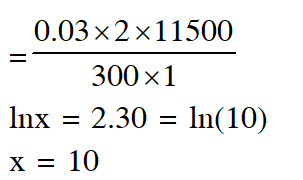Q. Consider an electrochemical cell: $\mathrm{A}(\mathrm{s})\left|\mathrm{A}^{\mathrm{n}+}(\mathrm{aq}, 2 \mathrm{M}) \| \mathrm{B}^{2 \mathrm{n}+}(\mathrm{aq}, 1 \mathrm{M})\right| \mathrm{B}(\mathrm{s})$. The value of $\Delta \mathrm{H}^{\theta}$ for the cell reaction is twice that of $\Delta \mathrm{G}^{\theta}$ at 300 K. If the emf of the cell is zero, the $\Delta \mathrm{S}^{\theta}$ $\left(\text { in } \mathrm{JK}^{-1} \mathrm{mol}^{-1}\right)$ of the cell reaction per mole of B formed at 300 K is___. (Given : ln (2) = 0.7, R (universal gas constant) = $8.3 \mathrm{JK}^{-1} \mathrm{mol}^{-1}$. H, S and G are enthalpy, entropy and Gibbs energy, respectively.) [JEE-Adv. 2018]
Ans. (-11.62)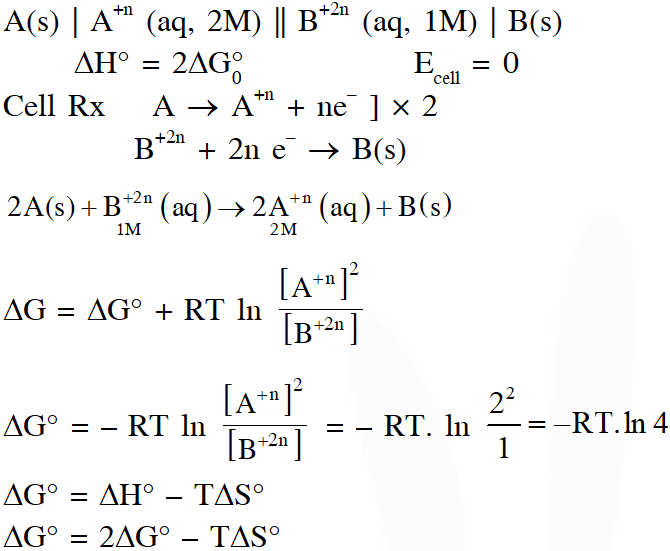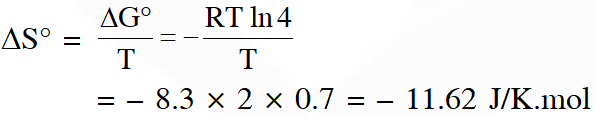Haripriya
May 4, 2023, 6:53 a.m.
Want more questions and witg videos solutions... Thank you 😊
lawde ka saral
Sept. 17, 2021, 7:51 a.m.
wahh betichod
Ddd
March 20, 2021, 2:20 p.m.
Super tankyou sooo much
hut
Nov. 20, 2020, 11:39 p.m.
xyz
THEHIYA
Oct. 10, 2020, 6:19 p.m.